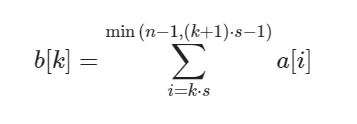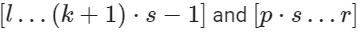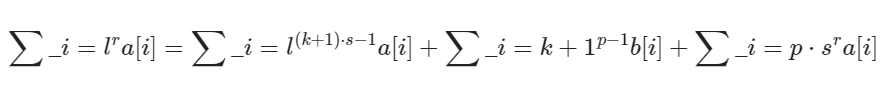New update is available. Click here to update.
Last Updated: May 24, 2023

# Mo's AlgorithmAuthor Saksham Gupta0 upvotes

## Introduction

If you are into sports then competitive programming is definitely for you, the only difference here is that it is more of a mind sport where you have to solve complex problems using complex algorithms. Today, we will discuss one such algorithm, i.e., Mo’s Algorithm, and see how it differs from the famous sqrt decomposition method.

## Sqrt Decomposition

Preprocessing is the basic concept of sqrt decomposition. We'll partition the array ‘a’ into blocks of roughly length sqrt(N) and precalculate the sum of the elements in each of the blocks. Let's say for a block, ‘k’ sum is b[k]. We will find the value of b[k] using the formula given below.As a result, we've figured out the values of b[k](this will cost us O(N) operations). But how can they assist us in answering the range queries [l,r]?

If the interval [l, r] is long enough, it will contain numerous full blocks, and we may find the sum of their elements in a single operation for those blocks. As a result, the interval will only contain parts of two blocks, and the sum of elements in these parts will be straightforward to calculate.

But how?

We only need sum of the two tail elements that areWe can use the formula below to solve the problemThese formulas must be very confusing for you. Don't worry. Once you look at its implementation, things will become easier.

### Code

``````#include <bits/stdc++.h>
using namespace std;

int main()
{
/*Input */
int n;
cin >> n;
vector<int> a(n, 0);
for (int i = 0; i < n; i++)
{
int x;
cin >> x;
a[i] = x;
}

/*Precalculations.*/
int sqrtValue = (int) sqrt(n + .0);

/*This is the size of one block */
int len = sqrtValue + 1;
vector<int> b;
b.resize(len);

/*Finding sums */
for (int i = 0; i < n; ++i)
b[i / len] += a[i];

int q;
cin >> q;

/*Queries*/
for (int i = 0; i < q; i++)
{
int l, r;
cin >> l >> r;

int sum = 0;
for (int i = l; i <= r;)
if (i + len - 1 <= r && i % len == 0)

{   /*If the complete block 'i' belongs to[l,r]*/
sum = sum + b[i / len];
i = i + len;
}
else
{
sum += a[i];
++i;
}
cout << sum << endl;
}
}``````

#### Input

``````9
1 1 2 1 3 4 5 2 8
3
0 4
1 3
2 4``````

#### Output

``````8
6
4``````

### Complexities

#### Time Complexity

O(Q * sqrt(N)), where ‘N’ is the size of the array and ‘Q’ is the number of queries.

Reason We are first pre-processing the sums that will cost us O(N) time then we for every query we will run a loop from ‘L’ to ‘R’ which even in the worst case will cost us O(sqrt(N)) time. Thus, the overall time complexity to O(Q * sqrt(N)) + O(N) ~ O(Q * sqrt(N)).

#### Space Complexity

O(N), where ‘N’ is the size of the array.

Reason: This is the space used by sum array ‘b’.

Read More - Time Complexity of Sorting Algorithms

## Mo’s Algorithm

We have seen how we can solve Range queries () using sqrt decomposition. Similar to sqrt decomposition, we have Mo's Algorithm. Now, we must precompute the answers for each block in a standard sqrt decomposition and merge them when answering queries. In some cases, the merging process can be rather difficult.
For example, if each query requests the mode of its range (the number that appears the most often). This would necessitate each block storing the count of each integer in some sort of data structure, and we can no longer conduct the merge step quickly enough.
Mo's method takes an entirely new approach to answer these types of questions quickly, as it only keeps track of one data structure and only performs simple and quick operations on it. The concept is to respond to questions in a specific order based on the indexes. All queries with the left index in block 0 will be answered first, followed by queries with the left index in block 1, and so on. Also, we'll have to respond to a block's questions in a specific sequence, specifically by sorting the queries by their right index. We will only use one data structure.
The information about the range will be stored in this data structure. This range will be empty at the start. When we want to answer the following inquiry (in the specific order), we simply add or remove elements on both sides of the current range until it becomes the query range. We just have to add or remove a single piece at a time this way, which should be relatively simple operations in our data structure.

But, This is only possible if we are allowed to answer queries in offline mode because we change the order in which they are answered.

Sounds a bit overwhelming? Let's look at a problem statement that will clear all of your doubts.

## Problem Statement

We are given an array and some queries. Our task is to find the sum of every query range.

Now the naive approach would be to loop over the given range(L, R) and find sum for every query. But we are not gonna use that here. We will see how we can use Mo’s algorithm to solve this problem. Let’s say that ‘ARR’ is given to us and its size is ‘N’.

MO's algorithm works by pre-processing all queries such that the results of one query can be used in the next. The steps are listed below.

• All queries should be sorted in such a way that queries with ‘L’ values ranging from 0 to sqrt(N) – 1 are grouped together, followed by queries with ‘L’ values ranging from n to 2 * sqrt(N) – 1, and so on. Within a block, all queries are ordered in increasing order of R values.
• Process each question one at a time, making sure that each one uses the sum computed in the preceding query.
• Let ‘SUM’ be the total sum of the previous queries.
• Remove any remaining entries from the preceding query. If the prior query was [0, 8], and the current query is [3, 9], we deduct ARR, ARR, and ARR from the ‘SUM’.
• To the current query, add new elements. We add a to total in the same example as before.

Let’s look at the implementation of the problem.

Attention reader!! Before looking at the implementation, you can try submitting the code here by yourself.

Implementation

``````#include <bits/stdc++.h>
using namespace std;

/*To store size of a particular block. */
int block;

/*Comparator function to sort queries */
bool compare(vector<int> x, vector<int> y)
{
/*Sorting by block*/
if (x / block != y / block)
return x / block < y / block;

/*Based on R values. */
return x < y;
}

void queryResults(vector<int> arr, vector<vector< int>> query)
{
block = (int) sqrt(arr.size());

/*Sorting queries. */
sort(query.begin(), query.begin() + query.size(), compare);

int currL = 0, currR = 0;
int currSum = 0;

/*Traversing through queries */
for (int i = 0; i < query.size(); i++)
{
int L = query[i], R = query[i];

/*Removing extra Elements*/
while (currL < L)
{
currSum -= arr[currL];
currL++;
}

/*Adding Elements that are in current Range */
while (currL > L)
{
currSum += arr[currL - 1];
currL--;
}

while (currR <= R)
{
currSum += arr[currR];
currR++;
}

/*Removing Elements of previous ranges */
while (currR > R + 1)
{
currSum -= arr[currR - 1];
currR--;
}

/*Printing the sum */
cout << "Sum of[" << L << ", " << R <<
"] is " << currSum << endl;
}
}

// Driver program
int main()
{
int n;
cin >> n;
vector<int> arr(n, 0);

/*Input */
for (int i = 0; i < n; i++)
{
int x;
cin >> x;
arr[i] = x;
}

int q;
cin >> q;
vector<vector < int>> queries;
for (int i = 0; i < q; i++)
{
int l, r;
cin >> l >> r;
queries.push_back({ l, r });

}

queryResults(arr, queries);
return 0;
}``````

#### Input

``````9
1 1 2 1 3 4 5 2 8
3
0 4
1 3
2 4``````

#### Output

``````Sum of [1, 3] is 4
Sum of [0, 4] is 8
Sum of [2, 4] is 6``````

### Complexities

#### Time Complexity

O((N + Q) * sqrt(N)), where ‘N’ is the size of the array and ‘Q’ is the number of queries.

Reason We can see that the index variable for ‘R’ changes at most O(N * sqrt(N) ) times even in the worst-case and similarly for ‘L’ it changes its value at most O(Q * sqrt(N)) times Thus, the overall time complexity to O(N * sqrt(N)) + O(Q * sqrt(N)) ~ O((N + Q) * sqrt(N)).

#### Space Complexity

O(1)

Reason: We are not using any external space.

## FAQs

1. What is meant by sqrt decomposition?
One of the most prevalent query optimization techniques used by competitive programmers is the sqrt (or Square Root) Decomposition Technique. We can cut Time Complexity by a factor of sqrt using this strategy (n). The main idea behind this technique is to break down a large array into little bits of size sqrt (n).

2. What types of problems can be solved using sqrt decomposition?
When there are many range queries on an array and changes to the array's elements, we can use it. This strategy should be used only when the number of update operations exceeds the number of query range operations; otherwise, segment trees can be used.

3. What is a segment tree?
A segment tree, also known as a statistic tree, is a data structure that stores information about intervals or segments. It allows you to see which segments in the database contain a specific point.

4. What types of problems can be solved using segment trees?
A large number of problems can be solved using segment trees, but segment trees are most used to solve problems in which we have to solve min, max, or sum queries of a given range.

5. Is there any other Data Structures and Algorithms content in CodeStudio?
Yes, CodeStudio allows you to practice coding as well as answer frequently asked interview questions. The more we practice, the more likely we are to acquire a job at our dream company.

## Key Takeaways

We saw how we could solve range query problems using Mo’s algorithm and solved the problem ‘Sum Range Query',.Some other problems that revolve around the Range query concepts are Range minimum queryMatrix range query, etc. Now don't waste any more time and head over to our practice platform CodeStudio to practice top problems on every topic, interview experiences, and many more. Till then, Happy Coding!

Codekaze-June23 India's Biggest Tech Hiring Challenge is LIVE!Go on top